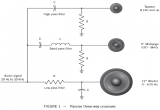# News Blogs

## Top 10 most Important schematic symbols for designing circuits

April 19, 2021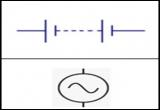We all are surrounded by electronic devices including phones, laptops, TV etc, but did you ever wonder what these things are made of. Most people might answer circuits which is correct to a point. A circuit is a closed path that allow electricity to flow from one end to another and made of various individual components such as resistors, capacitors, inductors etc.

## How to Calculate Wire Diameter & Cross-Sectional Area

December 22, 2020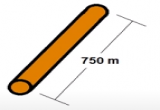In this blog, we will review the concept of resistance, resistivity and steps to calculate the minimum cross-sectional area and diameter of any desired conductor.

## What is Resistance?

The property of a device or a circuit that opposes the movement of current through it. Resistance is measured in Ohms (Ω). The resistance of any material with a uniform cross-sectional area is determined by the following four factors:

## An Overview of Magnetic Field Lines and its Characteristics

November 26, 2020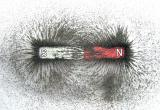In this blog, we will review the theory of Magnetic Fields and its basic concept. We all know that magnets exert forces on each other and its magnetic force is distributed around. Magnetic field lines are used to describe these forces around the magnet. We understand that magnets have two poles and that depending on the orientation of two magnets there can be an attraction (opposite poles) or repulsion (similar poles).

## Why Do We Need an Air Gap in Magnetic Circuit & How to Calculate it?

October 15, 2020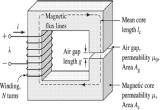In this blog we will review the theory of the Air gaps in magnetic circuits. A magnetic circuit is where a magnetic flux is circulated or follow through a closed area or path. An air gap is a non-magnetic part of a magnetic circuits and it is usually connected magnetically in series with the rest of the circuit. This allows a substantial part of the magnetic flux flows through the gap.

## Everything you need to know about current divider circuits

September 24, 2020In this blog we will review the theory of the Current Divider circuits in a parallel circuit. In a parallel circuit, all the components have their terminals connected together sharing the same two end nodes. This results in different paths and branches for the current to flow or pass along. However, the currents can have different values through each component but the voltage remains the same across two end nodes.

## The Importance of Inductance of a Coil

July 15, 2020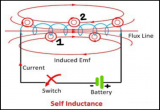In this blog we will explore Inductance; one of the major properties of an electrical circuit or an electronic device. Inductance is defined as a property of electrical circuit or a device that opposes a change in current. It is important to note that inductance does not oppose the current but rather opposes the change in current flowing within the circuit.